The QGradient class is used in combination with QBrush to specify gradient fills. More...

 Header: #include qmake: QT += gui Inherited By:

Detailed Description

Qt currently supports three types of gradient fills:

• Linear gradients interpolate colors between start and end points.
• Simple radial gradients interpolate colors between a focal point and end points on a circle surrounding it.
• Extended radial gradients interpolate colors between a center and a focal circle.
• Conical gradients interpolate colors around a center point.

A gradient's type can be retrieved using the type() function. Each of the types is represented by a subclass of QGradient: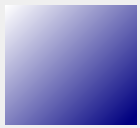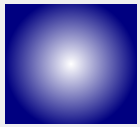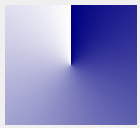The colors in a gradient are defined using stop points of the QGradientStop type; i.e., a position and a color. Use the setColorAt() function to define a single stop point. Alternatively, use the setStops() function to define several stop points in one go. Note that the latter function replaces the current set of stop points.

It is the gradient's complete set of stop points (accessible through the stops() function) that describes how the gradient area should be filled. If no stop points have been specified, a gradient of black at 0 to white at 1 is used.

A diagonal linear gradient from black at (100, 100) to white at (200, 200) could be specified like this:

A gradient can have an arbitrary number of stop points. The following would create a radial gradient starting with red in the center, blue and then green on the edges:

It is possible to repeat or reflect the gradient outside its area by specifiying the spread method using the setSpread() function. The default is to pad the outside area with the color at the closest stop point. The currently set spread method can be retrieved using the spread() function. The QGradient::Spread enum defines three different methods: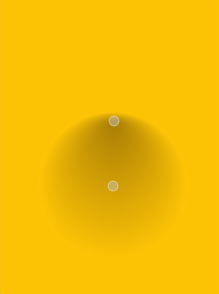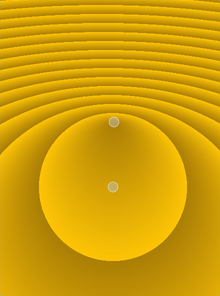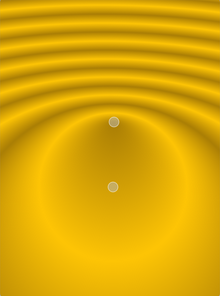PadSpread RepeatSpread ReflectSpread

Note that the setSpread() function only has effect for linear and radial gradients. The reason is that the conical gradient is closed by definition, i.e. the conical gradient fills the entire circle from 0 - 360 degrees, while the boundary of a radial or a linear gradient can be specified through its radius or final stop points, respectively.

The gradient coordinates can be specified in logical coordinates, relative to device coordinates, or relative to object bounding box coordinates. The coordinate mode can be set using the setCoordinateMode() function. The default is LogicalMode, where the gradient coordinates are specified in the same way as the object coordinates. To retrieve the currently set coordinate mode use coordinateMode().

Member Type Documentation

This enum specifies how gradient coordinates map to the paint device on which the gradient is used.

ConstantValueDescription
QGradient::LogicalMode0This is the default mode. The gradient coordinates are specified logical space just like the object coordinates.
QGradient::ObjectMode3In this mode the gradient coordinates are relative to the bounding rectangle of the object being drawn, with (0,0) in the top left corner, and (1,1) in the bottom right corner of the object's bounding rectangle. This value was added in Qt 5.12.
QGradient::StretchToDeviceMode1In this mode the gradient coordinates are relative to the bounding rectangle of the paint device, with (0,0) in the top left corner, and (1,1) in the bottom right corner of the paint device.
QGradient::ObjectBoundingMode2This mode is the same as ObjectMode, except that the {QBrush::transform()} {brush transform}, if any, is applied relative to the logical space instead of the object space. This enum value is deprecated and should not be used in new code.

This enum was introduced or modified in Qt 4.4.

ConstantValue

This enum was introduced or modified in Qt 5.12.

Specifies how the area outside the gradient area should be filled.

ConstantValueDescription

ConstantValueDescription

Member Function Documentation

Constructs a gradient based on a predefined preset.

The coordinate mode of the resulting gradient is QGradient::ObjectMode, allowing the preset to be applied to arbitrary object sizes.

This function was introduced in Qt 5.12.

Returns the coordinate mode of this gradient. The default mode is LogicalMode.

This function was introduced in Qt 4.4.

Creates a stop point at the given position with the given color. The given position must be in the range 0 to 1.

Sets the coordinate mode of this gradient to mode. The default mode is LogicalMode.

This function was introduced in Qt 4.4.

Note that this function only has effect for linear and radial gradients.

Replaces the current set of stop points with the given stopPoints. The positions of the points must be in the range 0 to 1, and must be sorted with the lowest point first.

Returns the stop points for this gradient.

If no stop points have been specified, a gradient of black at 0 to white at 1 is used.

Returns true if the gradient is the same as the other gradient specified; otherwise returns false.

This function was introduced in Qt 4.2.

Returns true if the gradient is the same as the other gradient specified; otherwise returns false.